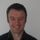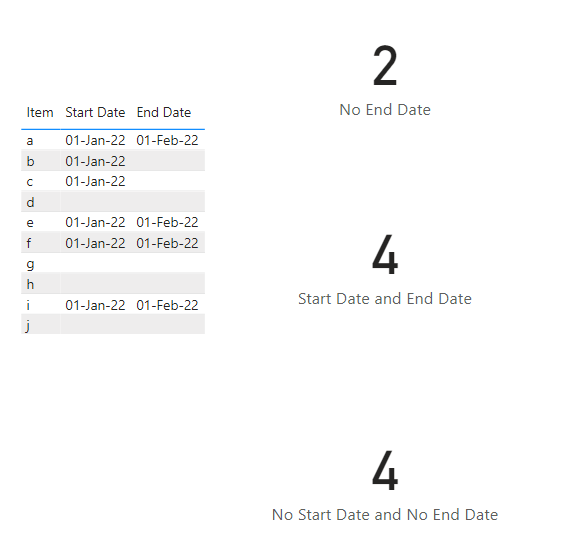cancel
Showing results for
Did you mean:Frequent Visitor

## Create columns that count number of blanks and populated cells

So I have two columns: "Start date" and "End date" Some cells in the columns, however, are blank. So, I want to create 3 columns that add a "1" in the their respective cells based on the following conditions:

Column One: If row has a start date and end date, then add a "1"

Column Two: If row has a start date and no end date, then add a "1"

Column Three: If row has no start date and no end date, then add a "1"

Reason I want three columns is because then I'll add up those 1s to display the number of rows that fall under each category.

1 ACCEPTED SOLUTIONSuper User

You can do this with measures.  Usually best to use measures when you can rather than create unnecessary columns in your data set.

``Start Date and End Date = CALCULATE(COUNTROWS('DataTable'), FILTER('DataTable', NOT ISBLANK('DataTable'[End Date]) && NOT ISBLANK('DataTable'[Start Date])))``

``No End Date = CALCULATE(COUNTROWS('DataTable'), FILTER('DataTable', ISBLANK('DataTable'[End Date]) && NOT ISBLANK('DataTable'[Start Date])))``

``No Start Date and No End Date = CALCULATE(COUNTROWS('DataTable'), FILTER('DataTable', ISBLANK('DataTable'[End Date]) && ISBLANK('DataTable'[Start Date])))``Regards

Phil

If I helped you, click on the Thumbs Up to give Kudos.

Proud to be a Super User!

3 REPLIES 3Community Support

Hi @pintobean87,

Did the above suggestions help with your scenario? if that is the case, you can consider Kudo or Accept the helpful suggestions to help others who faced similar requirements.

If these also don't help, please share more detailed information to help us clarify your scenario to test.

Regards,

Xiaoxin Sheng

Community Support Team _ Xiaoxin
If this post helps, please consider accept as solution to help other members find it more quickly.Community Champion
Try add three columns with the codes respectively:
Column One = IF ([Start Date]<>BLANK()&&[End Date]<>BLANK(),1)
Column Two = IF ([Start Date]<>BLANK()&&[End Date]=BLANK(),1)
Column Three = IF ([Start Date]=BLANK()&&[End Date]=BLANK(),1)

If you feel comfortable with Power Query, you shall turn to PQ to add conditional columns.Super User

You can do this with measures.  Usually best to use measures when you can rather than create unnecessary columns in your data set.

``Start Date and End Date = CALCULATE(COUNTROWS('DataTable'), FILTER('DataTable', NOT ISBLANK('DataTable'[End Date]) && NOT ISBLANK('DataTable'[Start Date])))``

``No End Date = CALCULATE(COUNTROWS('DataTable'), FILTER('DataTable', ISBLANK('DataTable'[End Date]) && NOT ISBLANK('DataTable'[Start Date])))``

``No Start Date and No End Date = CALCULATE(COUNTROWS('DataTable'), FILTER('DataTable', ISBLANK('DataTable'[End Date]) && ISBLANK('DataTable'[Start Date])))``Regards

Phil

If I helped you, click on the Thumbs Up to give Kudos.

Proud to be a Super User!